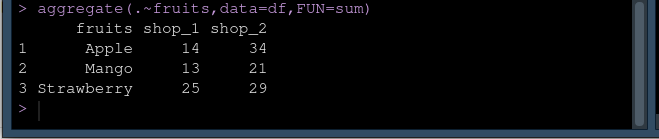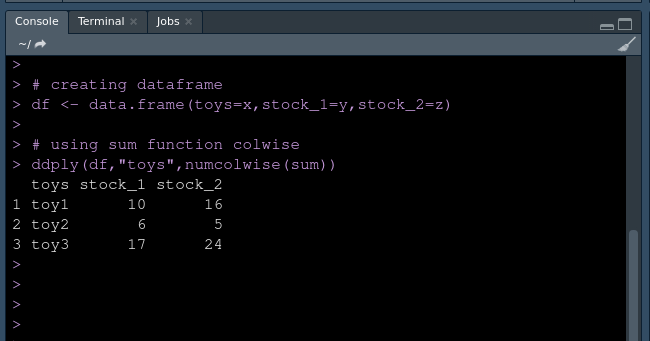# Sum of rows based on column value in R dataframe

• Last Updated : 01 Apr, 2021

In this article, we will be discussing how we can sum up row values based on column value in a data frame in R Programming Language. Suppose you have a data frame like this:

This dataset consists of fruits name and shop_1, shop_2 as a column name. Here shop_1 and shop_2 show the number of fruits available in shops. Now you want to find the aggregate sum of all the rows in shope_1 that have the same fruit value. So our dataset looks like this :

### Method 1: Using aggregate function

The aggregate function creates a subset of the original data and computes the statistical function for each subset and returns the result.

Syntax:

aggregate(.~fruit,data=df,FUN=sum)

Example:

## R

 `# Sum of rows based on column values`` ` `# Creating dataset ``# creating fuits column``x <- ``c``(``"Apple"``,``"Mango"``,``"Strawberry"``,``       ``"Apple"``,``"Apple"``,``"Strawberry"``,``       ``"Mango"``,``"Strawberry"``)`` ` `# creating shop_1 column``y <- ``c``(1,9,2,10,3,11,4,12)`` ` `# creating shop_2 column``z <- ``c``(13,5,14,6,15,7,16,8)`` ` `# creating dataframe``df <- ``data.frame``(fruits=x,shop_1=y,shop_2=z)`` ` `# applying aggregate function``aggregate``(.~fruits,data=df,FUN=sum)`

Output:Using aggregate function

### Method 2: Using ddply and  numcolwise function

ddply  simply split the given data frame and perform any  operation on it (probably apply a  function) and return the data frame.

colwise is a function from the famous plyr package. colwise function is used to compute a function on each column in data frame, it computes column wise.

Example:

## R

 `# Sum of rows based on column values`` ` `# loading library``library``(plyr)`` ` `# Creating dataset ``# creating fuits column``x <- ``c``(``"toy1"``,``"toy2"``,``"toy3"``,``       ``"toy1"``,``"toy1"``,``"toy3"``,``       ``"toy2"``,``"toy3"``)`` ` `# creating stock_1 column``y <- ``c``(1,2,3,4,5,6,4,8)`` ` `# creating stock_2 column``z <- ``c``(9,1,10,5,2,6,4,8)`` ` `# creating dataframe``df <- ``data.frame``(toys=x,stock_1=y,stock_2=z)`` ` `# using sum function colwise``ddply``(df,``"toys"``,``numcolwise``(sum))`

Output:Using ddply

My Personal Notes arrow_drop_up